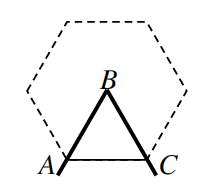### Home > CCG > Chapter 7 > Lesson 7.3.3 > Problem7-144

7-144.The angle created by a hinged mirror when forming a regular polygon is called a central angle. For example, $∠ABC$ in the diagram at right is the central angle of the regular hexagon.

1. If the central angle of a regular polygon measures $18°$, how many sides does the polygon have?

$\text{number}\ \text{of}\ \text{sides}=\frac{360}{18}$

2. Can a central angle measure $90°$? $180°$? $13°$? For each angle measure, explain how you know.

Try drawing shapes that could have the central angle measurements that have been listed.